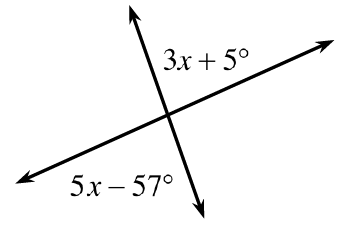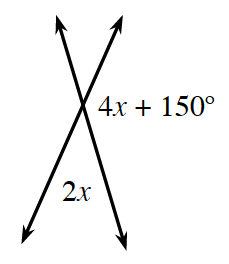### Home > CC3 > Chapter Ch9 > Lesson 9.2.7 > Problem9-151

9-151.

Examine the diagrams below. What is the geometric relationship between the labeled angles? What is the relationship of their measures? After you determine the relationship of their measures, use the relationship to write an equation and solve for $x$.

1.The two angles are vertical angles, which are always equal.

1.The angles are supplementary.

$x = 5º$